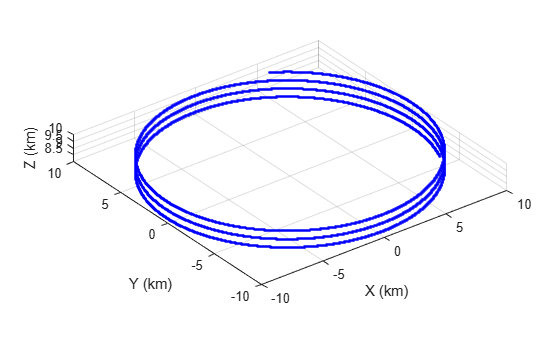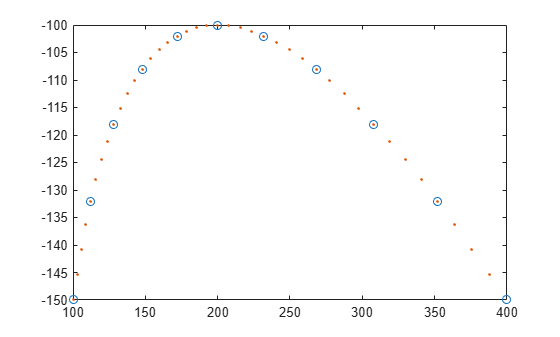# phased.Platform

Model platform motion

## Description

The `phased.Platform` System object™ models the translational motion of one or more platforms in space. A platform can be a target such as a vehicle or airplane, or a sonar or radar transmitter and receiver. The model assumes that the platform undergoes translational motion at constant velocity or constant acceleration during each simulation step. Positions and velocities are always defined in the global coordinate system.

To model a moving platform:

1. Define and set up your platform using the Construction procedure.

2. Repeatedly call the `step` method to move the platform along a path determined by the `phased.Platform` properties.

The behavior of `step` is specific to each object in the toolbox.

Note

Starting in R2016b, instead of using the `step` method to perform the operation defined by the System object, you can call the object with arguments, as if it were a function. For example, ```y = step(obj,x)``` and `y = obj(x)` perform equivalent operations.

## Construction

`sPlat = phased.Platform` creates a platform System object, `sPlat`. The object models a stationary platform with position at the origin and velocity set to zero.

`sPlat = phased.Platform(Name,Value)` creates an object, `sPlat`, with each specified property Name set to the specified Value. You can specify additional name-value pair arguments in any order as (`Name1`,`Value1`,...,`NameN`,`ValueN`).

`sPlat = phased.Platform(pos,vel,Name,Value)` creates a platform object, `sPlat`, with `InitialPosition` set to `pos` and `Velocity` set to `vel`. Other specified property Names are set to specified Values. The `pos` and `vel` arguments are value-only. Value-only arguments do not require a specified Name but are interpreted according to their argument positions. To specify any value-only argument, specify all preceding value-only arguments.

The motion model is either a constant velocity, a constant acceleration, or a custom trajectory. You can choose one of two motion models using the `MotionModel` property.

MotionModel ValueUsage
`'Velocity'`

If you set the `VelocitySource` property to `'Property'`, the platform moves with constant velocity determined by the `Velocity` property. You can specify the `InitialPosition` property or leave it to its default value. You can change the tunable `Velocity` property at any simulation step.

When you set the `VelocitySource` property to `'Input port'`, you can input instantaneous velocity as an argument to the `step` method. Specify the initial position using the `InitialPosition` property or leave it as a default value.

`'Acceleration'`

When you set the `AccelerationSource` property to `'Property'`, the platform moves with constant acceleration determined by the `Acceleration` property. You can specify the `InitialPosition` and `InitialVelocity` properties or leave them to their defaults. You can change the tunable `Acceleration` property at any simulation step.

When you set the `AccelerationSource` property to `'Input port'`, you can input instantaneous acceleration as an argument to the `step` method. Specify the `InitialPosition` and `InitialVelocity` properties or leave them as their defaults.

'Custom'Specify the platform motion using a series of waypoints in the `CustomTrajectory` property.

## Properties

 `MotionModel` Object motion model Object motion model, specified as `'Velocity'`, `'Acceleration'`, or `'Custom'`. When you set this property to `'Velocity'`, the platform follows a constant velocity trajectory during each simulation step. When you set this property to `'Acceleration'`, the platform follows a constant acceleration trajectory during each simulation step. When you set the property to `'Custom'`, the platform motion follows a sequence of waypoints specified by the `CustomTrajectory` property. The object performs a piecewise cubic interpolation on the waypoints to derive the position and velocity at each time step. Default: `'Velocity'` `InitialPosition` Initial position of platform Initial position of platform, specified as a real-valued 3-by-1 column vector in the form of `[x;y;z]` or a real-valued 3-by-N matrix where N is the number of platforms. Each column takes the form `[x;y;z]`. Position units are meters. Default: `[0;0;0]` `InitialVelocity` Initial velocity of platform Initial velocity of platform, specified as a real-valued 3-by-1 column vector in the form of `[vx;vy;vz]` or a real-valued 3-by-N matrix where N is the number of platforms. Each column taking the form `[vx;vy;vz]`. Velocity units are meters per second. This property only applies when you set the `MotionModel` property to `'Velocity'` and the `VelocitySource` to `'Input port'`, or when you set the `MotionModel` property to `'Acceleration'`. Default: `[0;0;0]` `VelocitySource` Source of velocity data Source of velocity data, specified as one of `'Property'` or ```'Input port'```. When you set the value of this property to `'Property'`, use `Velocity` property to set the velocity. When you set this property to `'Input port'`, use an input argument of the `step` method to set the velocity. This property applies when you set the `MotionModel` property to `'Velocity'`. Default: `'Property'` `Velocity` Current velocity of platform Specify the current velocity of the platform as a 3-by-1 real-valued column vector in the form of `[vx;vy;vz]` or a 3-by-N real-valued matrix for multiple platforms. Each column taking the form `[vx;vy;vz]`. Velocity units are meters/sec. The dimension N is the number of platforms. This property applies when you set the `MotionModel` property to `'Velocity'` and the `VelocitySource` to `'Property'`. This property is tunable. Default: `[0;0;0]` `AccelerationSource` Source of acceleration data Source of acceleration data, specified as one of `'Property'` or `'Input port'`. When you set the value of this property to `'Property'`, specify the acceleration using the `Acceleration` property. When you set this property to ```'Input port'```, use an input argument of the `step` method to set the acceleration. This property applies when you set the `MotionModel` property to `'Acceleration'`. Default: `'Property'` `Acceleration` Acceleration of platform Specify the current acceleration of the platform as a real-valued 3-by-1 column vector in the form `[ax;ay;az]` or a real-valued 3-by-N matrix with each column taking the form `[ax;ay;az]`. The dimension N is the number of platforms. Acceleration units are meters/sec/sec. This property applies when you set the `MotionModel` property to `'Acceleration'` and `AccelerationSource` to `'Property'`. This property is tunable. Default: `[0;0;0]` `CustomTrajectory` Custom trajectory waypoints. Custom trajectory waypoints, specified as a real-valued M-by-L matrix, or M-by-L-by-N array. M is the number of waypoints. L is either 4 or 7. When L is 4, the first column indicates the times at which the platform position is measured. Columns 2 - 4 are position measurements in x, y, and z coordinates. The velocity is derived from the position measurements.When L is 7, columns 5 - 7 in the matrix are velocity measurements in x, y, and z coordinates. When you set the `CustomTrajectory` property to a three-dimensional array, the number of pages, N, represent the number of platforms. Time units are in seconds, position units are in meters, and velocity units are in meters per second. To enable this property, set the `MotionModel` property to `'Custom'`. `ScanMode` Mechanical scanning mode Mechanical scan mode for platform, specified as `'None'`, `'Circular'`', or `'Sector'`, where `'None'` is the default. When you set the `ScanMode` property to `'Circular'`, the platform scan clockwise 360 degrees continuously in the azimuthal direction of the platform orientation axes. When you set the `ScanMode` property to `'Sector'`, the platform scans clockwise in the azimuthal direction in the platform orientation axes within a range specified by the `AzimuthSpan` property. When the platform scan reaches the span limits, the scan reverses direction and scans back to the other scan limit. Scanning happens within the orientation axes of the platform. `InitialScanAngle` Initial scan angle of platform Initial scan angle of platform, specified as a 1-by-N vector where N is the number of platforms. The scanning occurs in the local coordinate system of the platform. The `InitialOrientationAxes` specifies the original local coordinate system. At the start of the simulation, the orientation axes specified by the `InitialOrientationAxes` are rotated by the angle specified in the `InitialScanAngle` property. The default value is zero. Units are in degrees. This property applies when you set the `ScanMode` property to `'Circular'` or `'Sector'`. Example: [30 40] `AzimuthSpan` Azimuth span The azimuth angle span, specified as an N-by-2 matrix where N is the number of platforms. Each row of the matrix specifies the scan range of the corresponding platform in the form ```[ScanAngleLowerBound ScanAngleHigherBound]```. The default value is `[-60 60]`. Units are in degrees. To enable this property, set the `ScanMode` to `'Sector'`. `AzimuthScanRate` Azimuth scan rate Azimuth scan rate, specified as a 1-by-N vector where N is the number of platforms. Each entry in the vector is the azimuth scan rate for the corresponding platform. The default value is 10 degrees/second. Units are in degrees/second. To enable this property, set the `ScanMode` property to `'Circular'` or `'Sector'`. `InitialOrientationAxes` Initial orientation axes of platform Initial orientation axes of platform, specified as a 3-by-3 real-valued orthonormal matrix for a single platform or as a 3-by-3-by-N real-valued matrix for multiple platforms. The dimension N is the number of platforms. When the orientation matrix is 3-by-3, the three columns represent the axes of the local coordinate system (xyz). When the orientation matrix is 3-by-3-by-N, for each page index, the resulting 3-by-3 matrix represents the axes of a local coordinate system. Default: ```[1 0 0;0 1 0;0 0 1]``` `OrientationAxesOutputPort` Output orientation axes To obtain the instantaneous orientation axes of the platform, set this property to `true` and use the corresponding output argument when invoking `step`. If you do not want to obtain the orientation axes of the platform, set this property to `false`. Default: `false`

## Methods

 reset Reset platform to initial position step Output current position, velocity, and orientation axes of platform
Common to All System Objects
`release`

Allow System object property value changes

## Examples

collapse all

Create a platform at the origin having a velocity of (100,100,0) meters per second. Simulate the motion of the platform for two time steps, assuming the time elapsed for each step is one second. The position of the platform is updated after each step.

```sPlat = phased.Platform([0; 0; 0],[100; 100; 0]); T = 1;```

At the first call to step, the position is at its initial value.

```[pos,v] = step(sPlat,T); pos```
```pos = 3×1 0 0 0 ```

At the second call to step, the position changes.

```[pos,v] = step(sPlat,T); pos```
```pos = 3×1 100 100 0 ```

Start with an airplane moving along a circular track with a radius of 10 km at a horizontal speed of 100 m/s and descending at a rate of 1 m/sec. To create circular motion, specify a radially-inward acceleration and constrain the acceleration to lie in the horizontal plane. The acceleration of a body moving in a circle is $\frac{{\mathit{v}}^{2}}{\mathit{r}}$. The rate of descent is constant. Set the initial orientation axes matrix of the platform to the identity matrix.

Set up the initial conditions

```alt = 10000; radcirc = 10000; % 10 km phi = 60; initPos = [cosd(phi)*radcirc;sind(phi)*radcirc;alt]; vs = 100.0; vx = vs*sind(phi); vy = -vs*cosd(phi); vz = -1; initVel = [vx,vy,vz]'; airplane = phased.Platform('MotionModel','Acceleration', ... 'AccelerationSource','Input port','InitialPosition',initPos, ... 'InitialVelocity',initVel,'OrientationAxesOutputPort',true, ... 'InitialOrientationAxes',eye(3)); accelmag = vs^2/radcirc; initPos1 = [cosd(phi)*radcirc;sind(phi)*radcirc;0]; unitvec = initPos1/radcirc; accel = -accelmag*unitvec;```

Compute the trajectory

Compute the trajectory for 20000 integration steps at T = 0.1 s intervals

```N = 20000; tstep = .10; posmat = zeros(3,N); for n = 1:N [pos,vel,oax] = airplane(tstep,accel); velcirc2 = vel(1)^2 + vel(2)^2; vmag = sqrt(velcirc2); pos1 = [pos(1),pos(2),0]'; radcirc = sqrt(pos1'*pos1); unitvec = pos1/radcirc; accelmag = velcirc2/radcirc; accel = -accelmag*unitvec; posmat(:,n) = pos; end```

Display the final orientation of the local coordinate system.

`disp(oax)`
``` 0.1271 0.9919 0.0001 -0.9919 0.1271 0.0003 0.0003 -0.0001 1.0000 ```

Plot the trajectory

```plot3(posmat(1,:)/1000,posmat(2,:)/1000,posmat(3,:)/1000,'b.') xlabel('X (km)') ylabel('Y (km)') zlabel('Z (km)') axis equal grid```This example shows

Create waypoints from parabolic motion.

```x0 = 100; y0 = -150; z0 = 0; vx = 5; vy = 10; vz = 0; ax = 1; ay = -1; t = [0:2:20]; x = x0 + vx*t + ax/2*t.^2; y = y0 + vy*t + ay/2*t.^2; z = z0*ones(size(t)); wpts = [t.' x.' y.' z.'];```

Create a platform object with motion determined using waypoints.

```pltfm = phased.Platform('MotionModel','Custom','CustomTrajectory',wpts); tstep = .5; nsteps = 40; X = [];```

Advance the platform in time steps of one half second;.

```for k = 1:nsteps [pos,vel] = pltfm(tstep); X = [X;pos']; end plot(x,y,'o'); hold on plot(X(:,1),X(:,2),'.') hold off;```